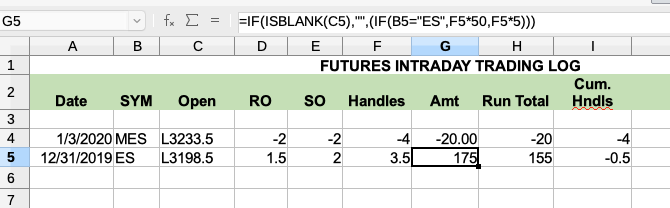# Formula changed by value in another cell?

Is there a way to set up a formula that changes based on the value in another cell?

I have a summation cell in column G, but I want the value to be calculated differently based on the symbol in column B. Right now I’m doing it with a conditional IF formula, but that only gives me two options. Since I want to use 5 or 6 different symbols appearing in column B, I’m looking for a way to automate that calculation which is different for each symbol.

Many thanks for any suggestions or guidance.Hello,

take a look at `IFS()` function here: LibreOffice Help - IFS, which is designed to avoid nesting of `IF` functions, which would be quite confusing on a 6-case distinction of your use case scenario.

Meta formula for your case: `=IF(ISBLANK(C5);"";IFS(B5="ES";F5*50;B5="MES";F5*4;B5=<NEXTC1>;<NEXTFORM1>....))`

Hope that helps.

If the answer helped you to solve your problem, please click the check mark () next to the answer.

I think that first IF can also be merged into IFS…

@mikekaganski - of course `=IFS(ISBLANK(C5);"";B5="ES";F5*50;B5="MES";F5*4;B5=<NEXTC1>;<NEXTFORM1>....)` should be the same (did not test; I’m too lazy now to typewrite the image data ;-))

That’s it! Many thanks!